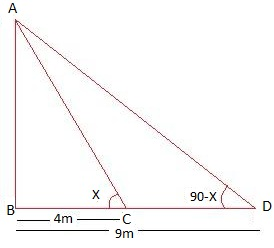Guru

# The angles of elevation of the top of a tower from two points at a distance of 4 m and 9 m from the base of the tower and in the same straight line with it are complementary. Prove that the height of the tower is 6 m. Q.16

• 0

How i solve this problem in easy way of question from Some applications of Trigonometry of exercise no.9.1 of class 10th ncert math, sir please help me the best way to solve this problem The angles of elevation of the top of a tower from two points at a distance of 4 m and 9 m from the base of the tower and in the same straight line with it are complementary. Prove that the height of the tower is 6 m

Share

1. Let AB be the tower. C and D be the two points with distance 4 m and 9 m from the base respectively. As per question,In right ΔABC,

tan x = AB/BC

tan x = AB/4

AB = 4 tan x … (i)

Again, from right ΔABD,

tan (90°-x) = AB/BD

cot x = AB/9

AB = 9 cot x … (ii)

Multiplying equation (i) and (ii)

AB2 = 9 cot x × 4 tan x

⇒ AB2 = 36 (because cot x = 1/tan x

⇒ AB = ± 6

Since height cannot be negative. Therefore, the height of the tower is 6 m.

Hence Proved.

• 0